# Plot grid in R

There exists three main ways to add a grid to a plot in base R: using `grid`, `axis` or `abline` functions.

## The `grid` function

The `grid` function is specifically designed to add grids to an existing plot. The function allows modifying the number of sections where to divide each axis with the `nx` and `ny` arguments, e.g. `nx = 2` will create only a vertical line. You can also modify the color, width and type of the lines with the `col`, `lwd` and `lty` arguments, respectively.

Option 1. Add grid to X and Y axis.

``````# Data
set.seed(132)
x <- rnorm(500)

plot(x, pch = 19, col = 4)
grid(nx = NULL, ny = NULL,
lty = 2,      # Grid line type
col = "gray", # Grid line color
lwd = 2)      # Grid line width``````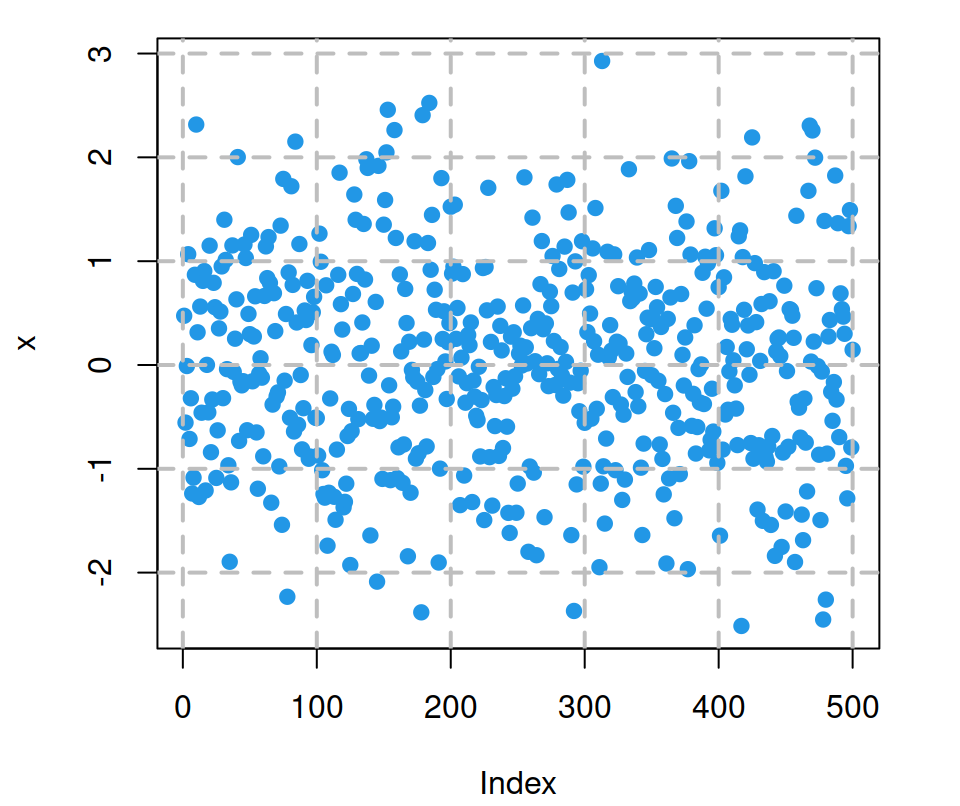Option 2. Add grid only to Y-axis.

``````# Data
set.seed(132)
x <- rnorm(500)

plot(x, pch = 19, col = 4)
grid(nx = NA,
ny = NULL,
lty = 2, col = "gray", lwd = 2)``````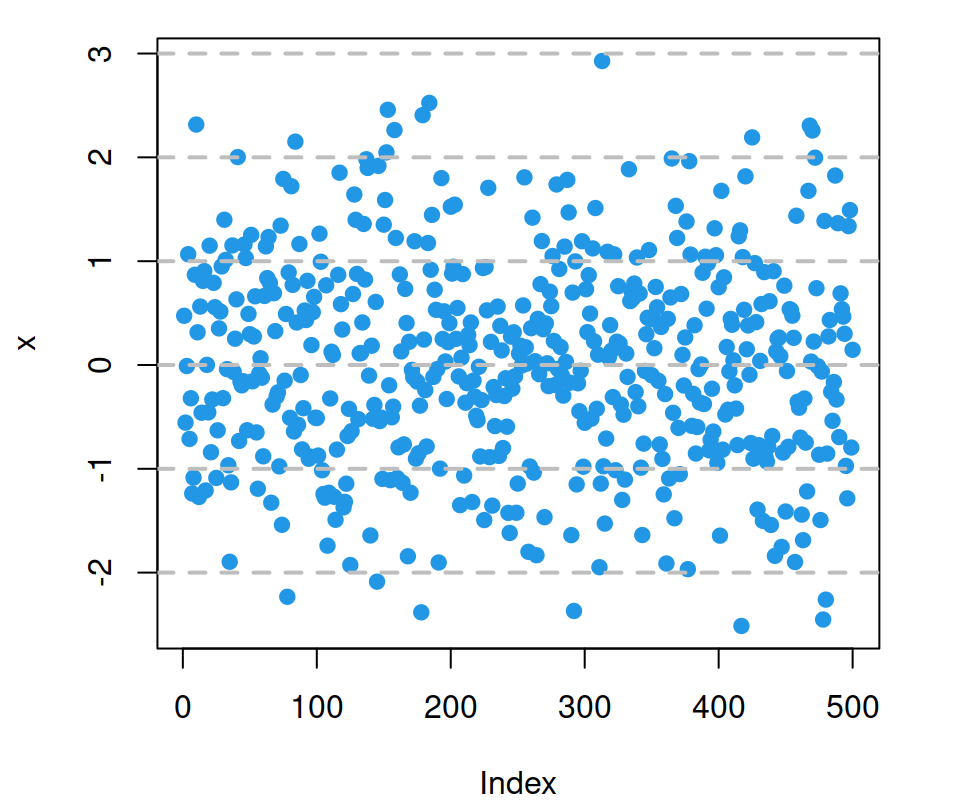Option 3. Add grid only to X-axis.

``````# Data
set.seed(132)
x <- rnorm(500)

plot(x, pch = 19, col = 4)
grid(nx = NULL,
ny = NA,
lty = 2, col = "gray", lwd = 2)``````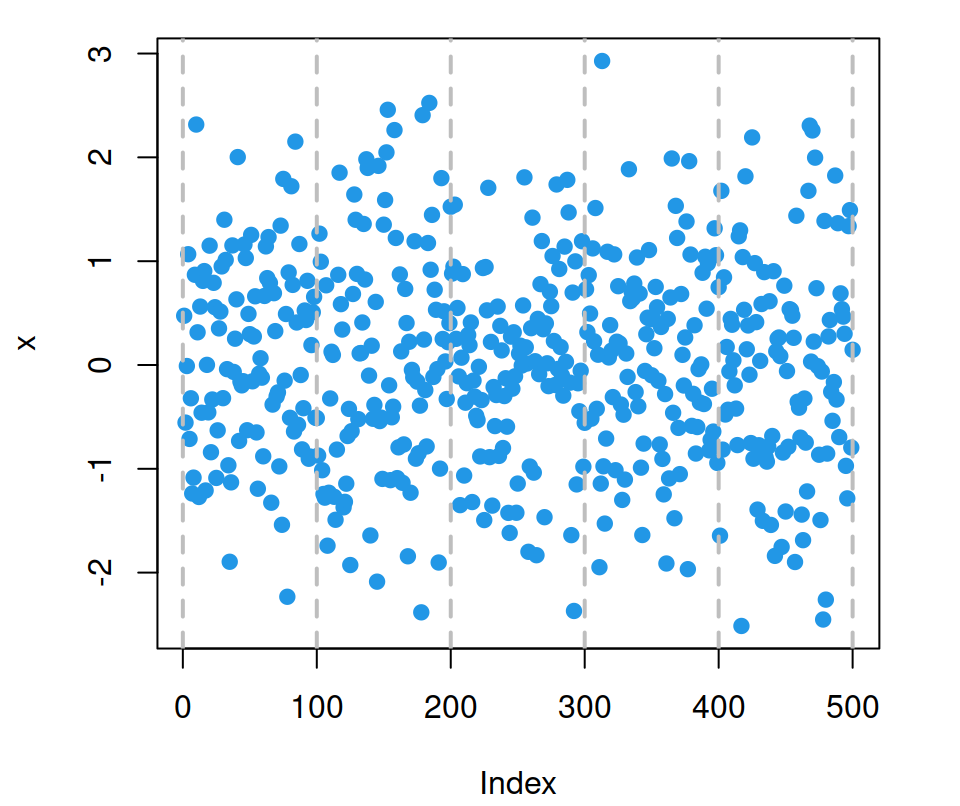Option 4. Customize the number of division sections with `nx` and `ny` arguments.

``````# Data
set.seed(132)
x <- rnorm(500)

plot(x, pch = 19, col = 4)
grid(nx = 2, # X-axis divided in two sections
ny = 3, # Y-axis divided in three sections
lty = 2, col = "gray", lwd = 2)``````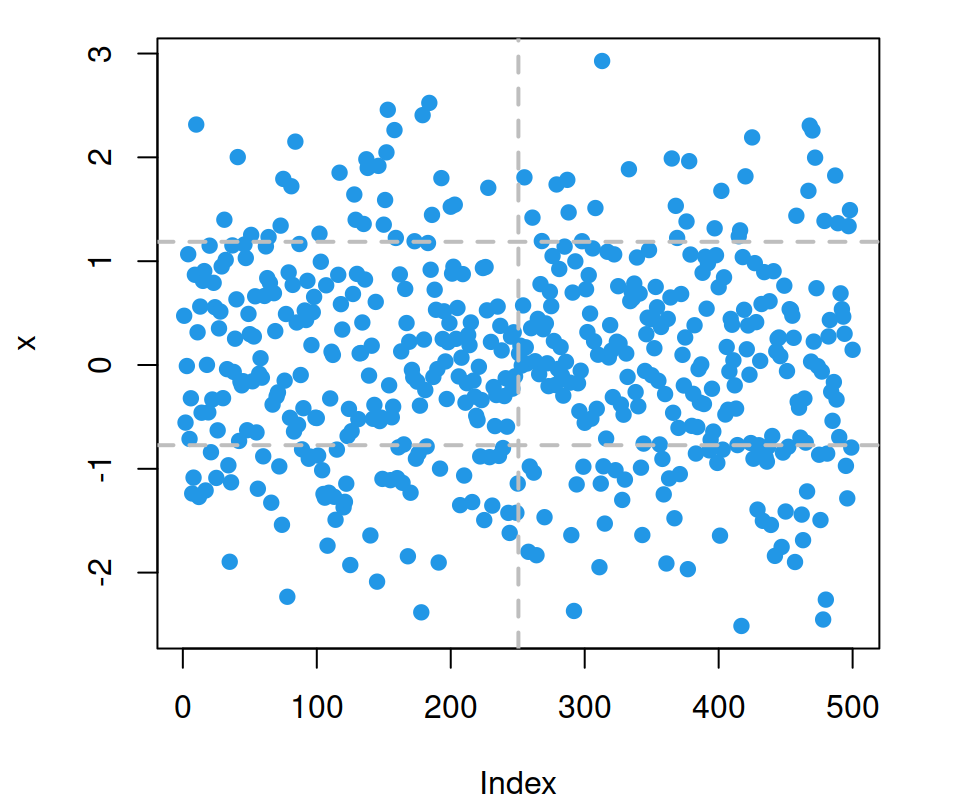### Grid below plot

You may have noticed that the grid is added over the plot. In case you want to have the grid below your plot you have to add your plot over.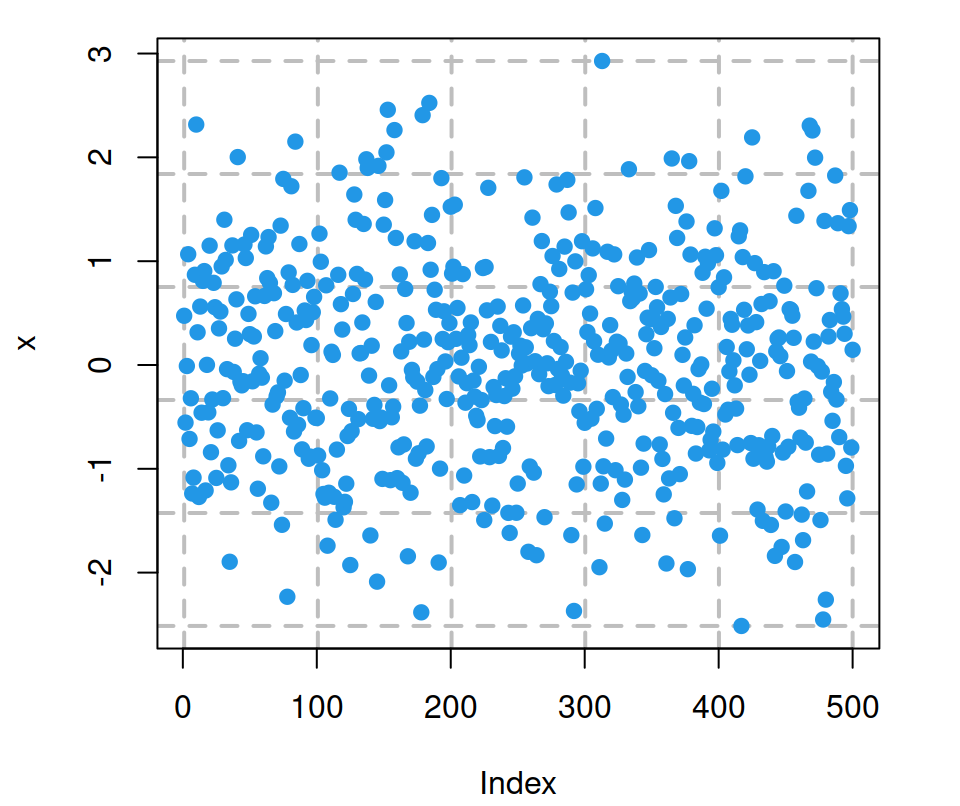Option 1. You can use `plot.new()` and `par(new = TRUE)`. However, the grid won’t match the axis ticks.

``````# Data
set.seed(132)
x <- rnorm(500)

plot.new()

grid(nx = NULL, ny = NULL,
lty = 2, col = "gray", lwd = 2)

par(new = TRUE)
plot(x, pch = 19, col = 4)``````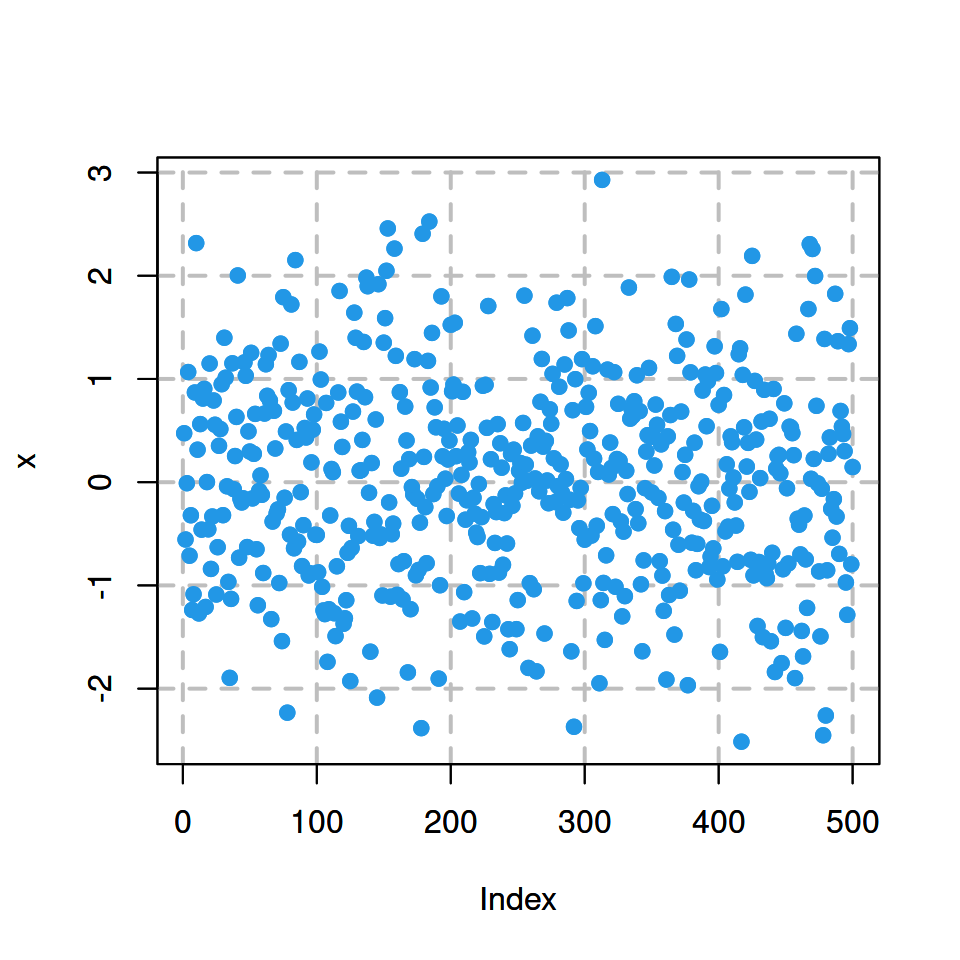Option 2. Instead of using `plot.new()` you can use the plotting function to plot your data:

``````# Data
set.seed(132)
x <- rnorm(500)

plot(x)

grid(nx = NULL, ny = NULL,
lty = 2, col = "gray", lwd = 2)

par(new = TRUE)
plot(x, pch = 19, col = 4)``````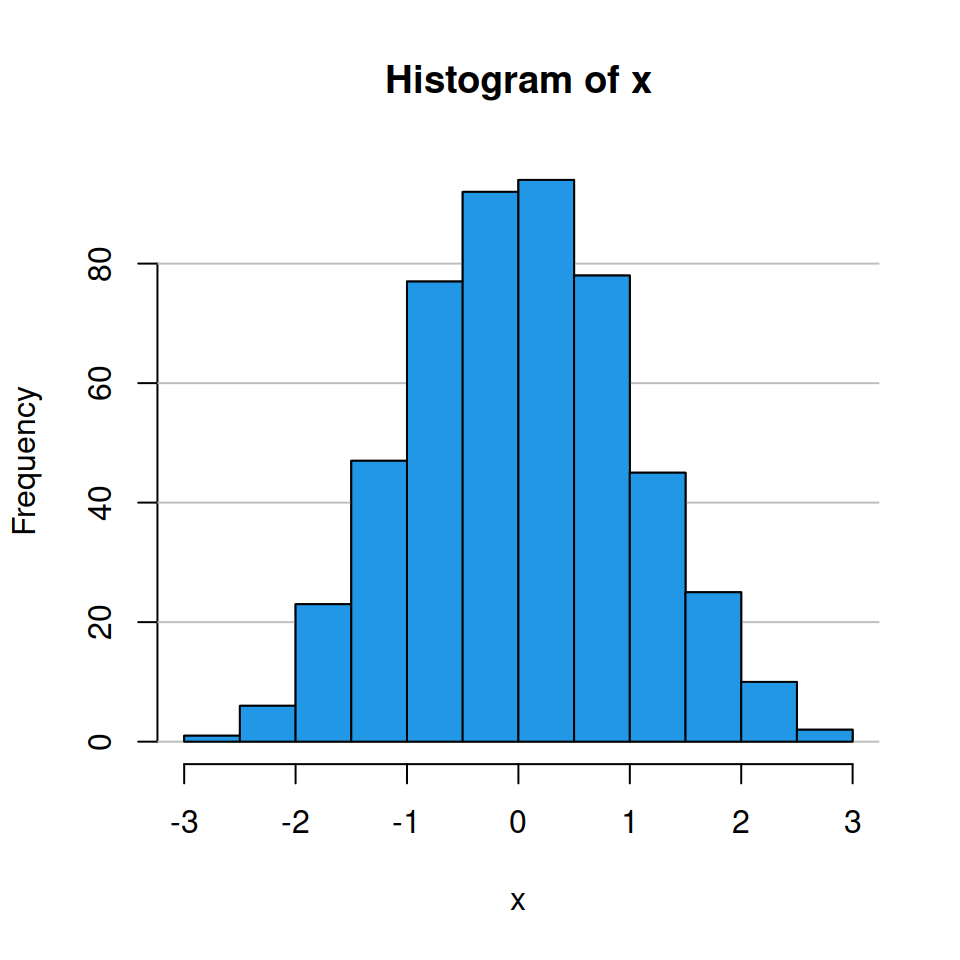Option 3. If the plotting function you are using (like `hist`, `boxplot` or `barplot`) supports the argument `add`, set `add = TRUE` instead of `par(new = TRUE)`.

``````# Data
set.seed(132)
x <- rnorm(500)

hist(x)
grid(nx = NA, ny = NULL,
lty = 1, col = "gray", lwd = 1)
hist(x, col = 4,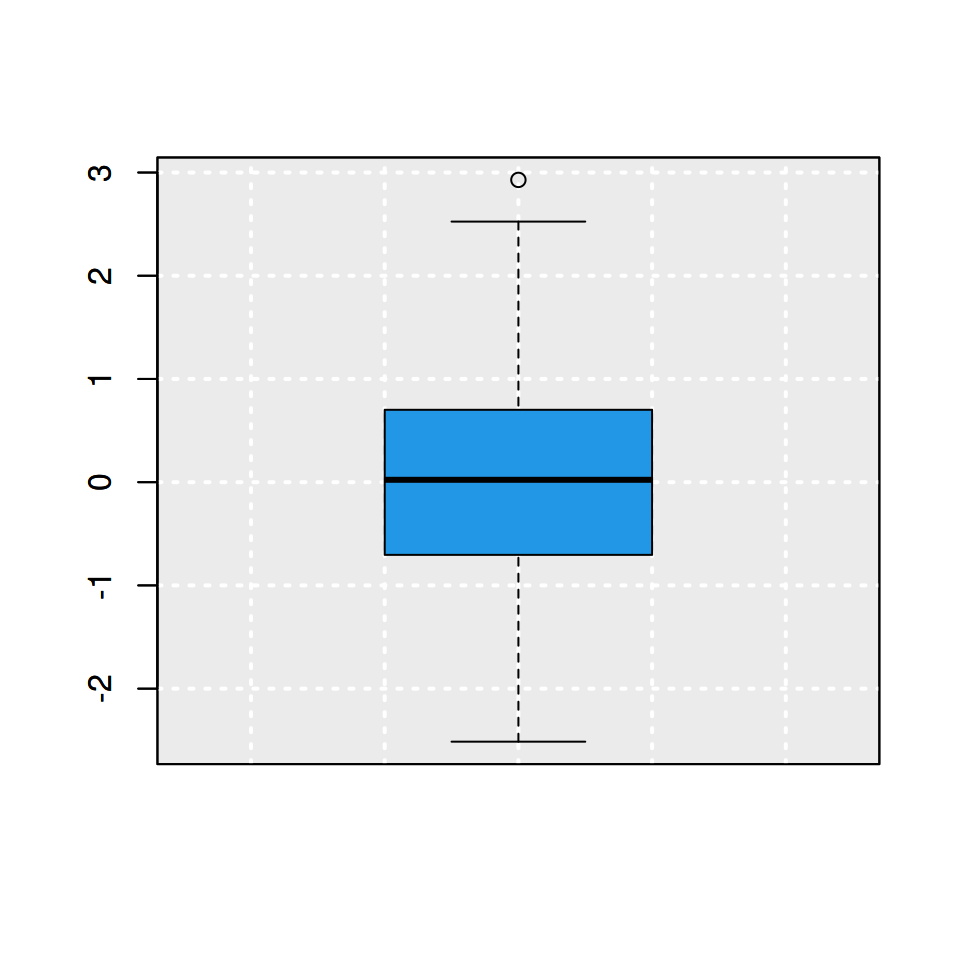Note that you can add a background color and a grid at the same time as follows:

``````# Data
set.seed(132)
x <- rnorm(500)

boxplot(x)

# Light gray background
rect(par("usr"), par("usr"),
par("usr"), par("usr"),
col = "#ebebeb")

grid(nx = NULL, ny = NULL,
col = "white", lwd = 2)

# Boxplot
boxplot(x, col = 4, add = TRUE)``````

If your chart is a scatter plot you can add the points over the grid with the `points` function.

## Adding a grid with the `axis` function

The `axis` function allows customizing the axes of the plots. However, if you set `tck = 1` the tick marks of the axes will create a grid.

``````# Data
set.seed(132)
x <- rnorm(500)

plot(x, pch = 19, col = 4)

# Vertical grid
axis(1, tck = 1, lty = 2, col = "gray")

# Horizontal grid
axis(2, tck = 1, lty = 2, col = "gray")

# Grid below plot
par(new = TRUE)
plot(x, pch = 19, col = 4)

# Box of the plot
box()``````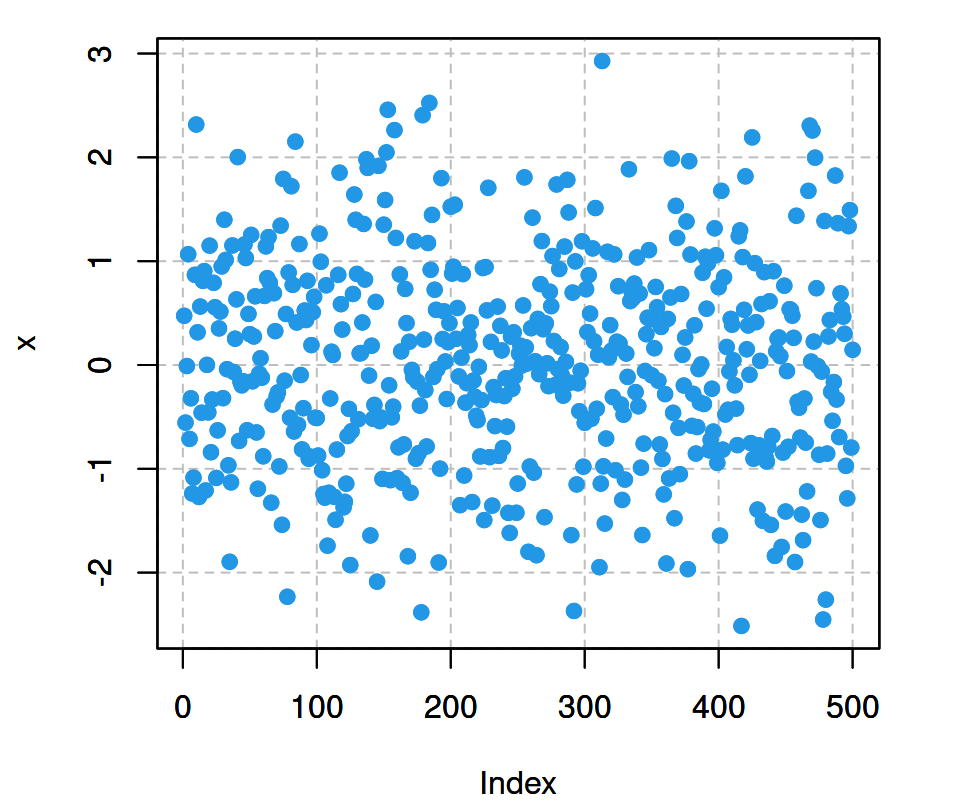## Grid with `abline` function

Similarly to `axis`, `abline` allows you to add grid lines at some values. The main benefit of this approach is that it doesn’t override the axis line, so you don’t have to use the `box` function to solve it.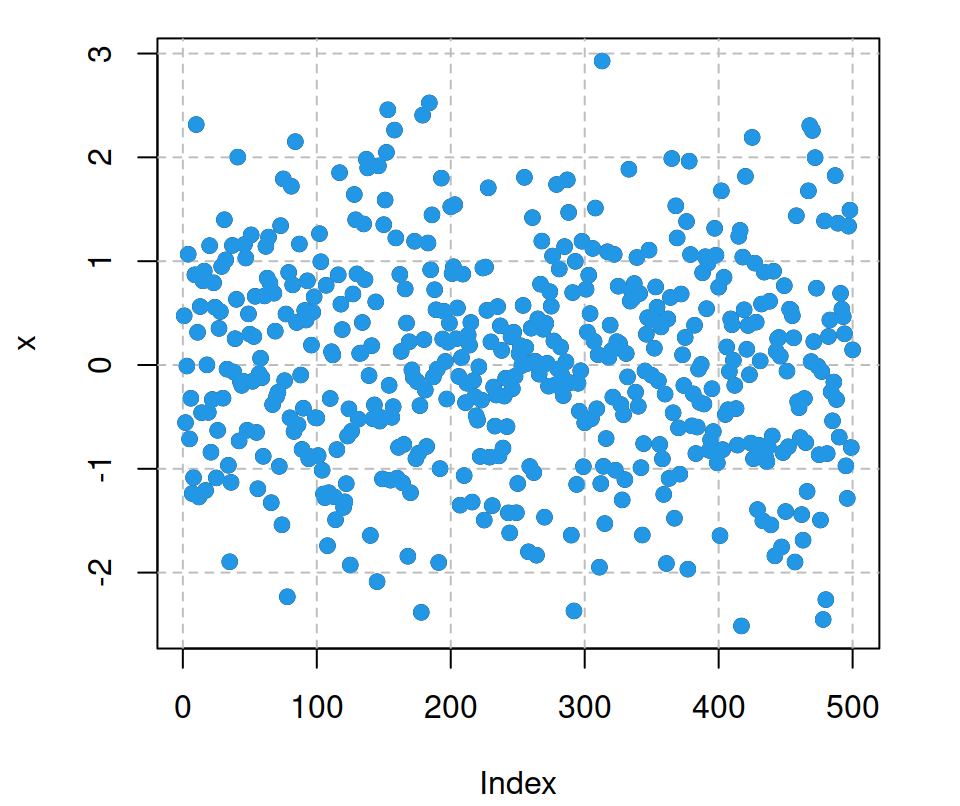``````# Data
set.seed(132)
x <- rnorm(500)

plot(x)

# Vertical grid
abline(v = seq(0, 500, 100),
lty = 2, col = "gray")

# Horizontal grid
abline(h = seq(-3, 3, 1),
lty = 2, col = "gray")

# Grid below points
points(x, pch = 19, col = 4)``````

## Align grid to ticks

When the ticks of the plot are different from the default you can align them using the `axis` or `abline` functions in case that specifying `nx` or `ny` arguments of the `grid` function doesn’t align them.

``````# Data
set.seed(132)
x <- rnorm(500)

plot(x,
xaxp = c(100, 400, 10),
yaxp = c(-2, 2, 5))

# Vertical grid
axis(1,
at = seq(100, 400, by = 20),
tck = 1, lty = 2, col = "gray")

# Horizontal grid
axis(2,
at = seq(-2, 2, by = 0.8),
tck = 1, lty = 2, col = "gray")

# Box of the plot
box()

# Grid below points
points(x, pch = 19, col = 4)``````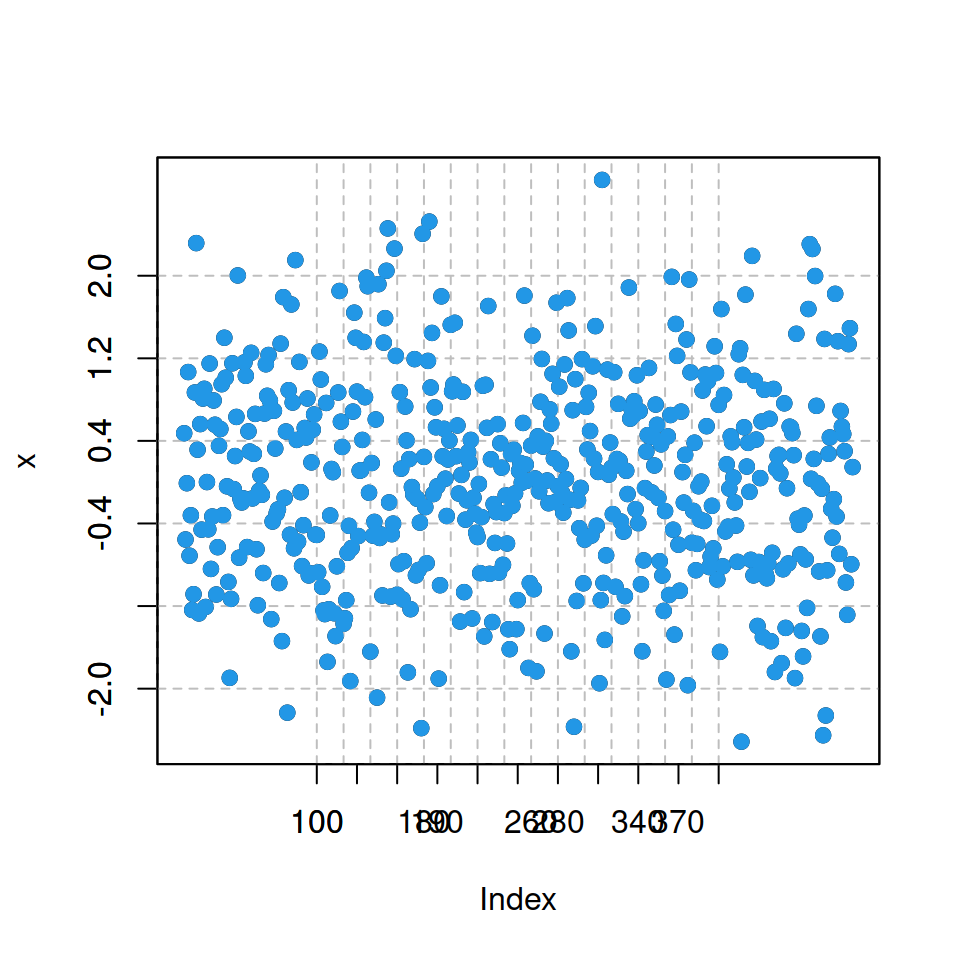The same can be achieved making use of `abline` instead of `axis`.